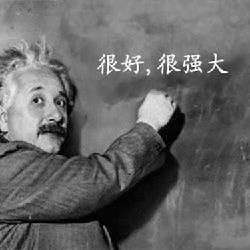# Java 8 Stream流详细用法

2022年3月15日Stream 究竟是什么呢？

Stream 就好像一个高级的迭代器，但只能遍历一次，就好像一江春水向东流；在流的过程中，对流中的元素执行一些操作，比如“过滤掉长度大于 10 的字符串”、“获取每个字符串的首字母”等。

1）中间操作，可以有多个，每次返回一个新的流，可进行链式操作。

2）终端操作，只能有一个，每次执行完，这个流也就用光光了，无法执行下一个操作，因此只能放在最后。

``````List<String> list = new ArrayList<>();

long count = list.stream().distinct().count();
System.out.println(count);
``````

`distinct()` 方法是一个中间操作（去重），它会返回一个新的流（没有共同元素）。

``````Stream<T> distinct();
``````

`count()` 方法是一个终端操作，返回流中的元素个数。

``````long count();
``````

## # 01、创建流

``````public class CreateStreamDemo {
public static void main(String[] args) {
String[] arr = new String[]{"武汉加油", "中国加油", "世界加油"};
Stream<String> stream = Arrays.stream(arr);

stream = Stream.of("武汉加油", "中国加油", "世界加油");

List<String> list = new ArrayList<>();
stream = list.stream();
}
}
``````

``````public static<T> Stream<T> of(T... values) {
return Arrays.stream(values);
}
``````

``````List<Long> aList = new ArrayList<>();
Stream<Long> parallelStream = aList.parallelStream();
``````

## # 02、操作流

Stream 类提供了很多有用的操作流的方法，我来挑一些常用的给你介绍一下。

### # 1）过滤

``````public class FilterStreamDemo {
public static void main(String[] args) {
List<String> list = new ArrayList<>();
Stream<String> stream = list.stream().filter(element -> element.contains("王"));
stream.forEach(System.out::println);
}
}
``````

`filter()` 方法接收的是一个 Predicate（Java 8 新增的一个函数式接口，接受一个输入参数返回一个布尔值结果）类型的参数，因此，我们可以直接将一个 Lambda 表达式传递给该方法，比如说 `element -> element.contains("王")` 就是筛选出带有“王”的字符串。

`forEach()` 方法接收的是一个 Consumer（Java 8 新增的一个函数式接口，接受一个输入参数并且无返回的操作）类型的参数，`类名 :: 方法名`是 Java 8 引入的新语法，`System.out` 返回 PrintStream 类，println 方法你应该知道是打印的。

`stream.forEach(System.out::println);` 相当于在 for 循环中打印，类似于下面的代码：

``````for (String s : strs) {
System.out.println(s);
}
``````

``````王力宏
``````

### # 2）映射

``````public class MapStreamDemo {
public static void main(String[] args) {
List<String> list = new ArrayList<>();
Stream<Integer> stream = list.stream().map(String::length);
stream.forEach(System.out::println);
}
}
``````

`map()` 方法接收的是一个 Function（Java 8 新增的一个函数式接口，接受一个输入参数 T，返回一个结果 R）类型的参数，此时参数 为 String 类的 length 方法，也就是把 `Stream<String>` 的流转成一个 `Stream<Integer>` 的流。

``````3
3
2
3
``````

### # 3）匹配

Stream 类提供了三个方法可供进行元素匹配，它们分别是：

• `anyMatch()`，只要有一个元素匹配传入的条件，就返回 true。

• `allMatch()`，只有有一个元素不匹配传入的条件，就返回 false；如果全部匹配，则返回 true。

• `noneMatch()`，只要有一个元素匹配传入的条件，就返回 false；如果全部匹配，则返回 true。

``````public class MatchStreamDemo {
public static void main(String[] args) {
List<String> list = new ArrayList<>();

boolean  anyMatchFlag = list.stream().anyMatch(element -> element.contains("王"));
boolean  allMatchFlag = list.stream().allMatch(element -> element.length() > 1);
boolean  noneMatchFlag = list.stream().noneMatch(element -> element.endsWith("沉"));
System.out.println(anyMatchFlag);
System.out.println(allMatchFlag);
System.out.println(noneMatchFlag);
}
}
``````

``````true
true
true
``````

### # 4）组合

`reduce()` 方法的主要作用是把 Stream 中的元素组合起来，它有两种用法：

• `Optional<T> reduce(BinaryOperator<T> accumulator)`

• `T reduce(T identity, BinaryOperator<T> accumulator)`

``````public class ReduceStreamDemo {
public static void main(String[] args) {
Integer[] ints = {0, 1, 2, 3};
List<Integer> list = Arrays.asList(ints);

Optional<Integer> optional = list.stream().reduce((a, b) -> a + b);
Optional<Integer> optional1 = list.stream().reduce(Integer::sum);
System.out.println(optional.orElse(0));
System.out.println(optional1.orElse(0));

int reduce = list.stream().reduce(6, (a, b) -> a + b);
System.out.println(reduce);
int reduce1 = list.stream().reduce(6, Integer::sum);
System.out.println(reduce1);
}
}
``````

``````6
6
12
12
``````

0、1、2、3 在没有起始值相加的时候结果为 6；有起始值 6 的时候结果为 12。

## # 03、转换流

``````public class CollectStreamDemo {
public static void main(String[] args) {
List<String> list = new ArrayList<>();

String[] strArray = list.stream().toArray(String[]::new);
System.out.println(Arrays.toString(strArray));

List<Integer> list1 = list.stream().map(String::length).collect(Collectors.toList());
List<String> list2 = list.stream().collect(Collectors.toCollection(ArrayList::new));
System.out.println(list1);
System.out.println(list2);

String str = list.stream().collect(Collectors.joining(", ")).toString();
System.out.println(str);
}
}
``````

`toArray()` 方法可以将流转换成数组，你可能比较好奇的是 `String[]::new`，它是什么东东呢？来看一下 `toArray()` 方法的源码。

``````<A> A[] toArray(IntFunction<A[]> generator);
``````

``````String[] strArray = (String[])list.stream().toArray((x\$0) -> {
return new String[x\$0];
});
System.out.println(Arrays.toString(strArray));
``````

``````List<Integer> list1 = list.stream().map(String::length).collect(Collectors.toList());
``````

Collectors 是一个收集器的工具类，内置了一系列收集器实现，比如说 `toList()` 方法将元素收集到一个新的 `java.util.List` 中；比如说 `toCollection()` 方法将元素收集到一个新的 ` java.util.ArrayList` 中；比如说 `joining()` 方法将元素收集到一个可以用分隔符指定的字符串中。

``````[周杰伦, 王力宏, 陶喆, 林俊杰]
[3, 3, 2, 3]
[周杰伦, 王力宏, 陶喆, 林俊杰]

``````